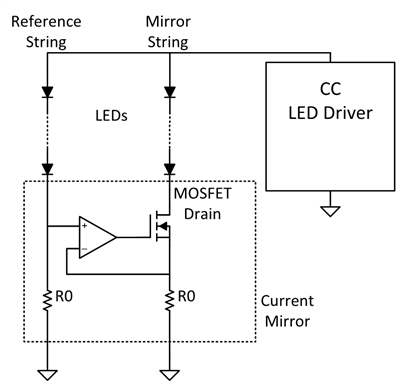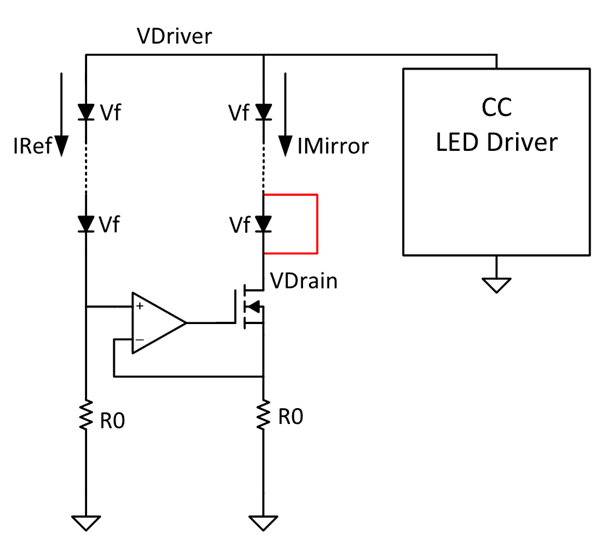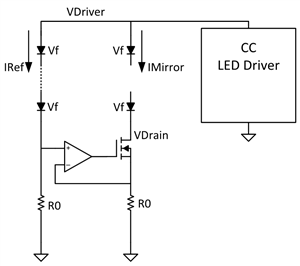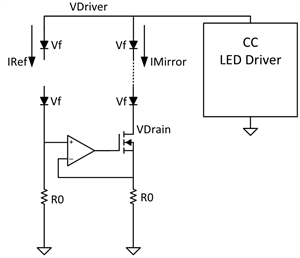# Designing daytime running lights, part 1: adding one-fail-all-fail LED fault detection

Daytime running lights (DRLs) are becoming more popular in vehicles and are even a requirement in some countries. As I’m sure you can guess, DRLs are on during the day, which requires them to be very bright.

As a designer, you typically have two choices to achieve the desired DRL LED brightness:

• Increase the number of LEDs in your string, which results in a much higher LED driver output voltage.
• Use two LED drivers to enable a lower LED driver output voltage.

Automotive Daytime Running Light Dual String LED Driver Reference Design with Current Balancing outlines an effective way to drive parallel strings of LEDs for DRL applications without adding a second LED driver. In the first installment of this two-part series, I’ll take a brief look at this reference design and investigate how to add one-fail-all-fail LED fault detection.

Reference design overview

The DRL reference design uses the current-balancing circuit shown in Figure 1 and the TPS92692-Q1 LED controller, which allows the device to drive parallel strings of LEDs using a single constant-current output. Equal current flows through both LED strings. Note that the reference string must have one more LED than the mirror string to properly bias the metal-oxide semiconductor field-effect transistor (MOSFET). The reference design guide describes the functionality of the current-balancing circuit in more detail.Figure 1: Current-balancing circuit

One-fail-all-fail LED fault detection

The main purpose of one-fail-all-fail LED fault detection is straightforward: if a single LED is shorted or opened in either the reference or mirror string, all LEDs in both strings will shut off. Although the concept is simple, its implementation requires a bit more thought and brings up two major questions:

• How is a fault detected?
• If a fault occurs, how do you shut off all of the LEDs?

How to detect a fault

While not obvious, it’s possible to detect a fault by monitoring the MOSFET drain voltage. There are four different potential types of faults:

• An LED short in the mirror string.
• An LED short in the reference string.
• An open circuit in the mirror string.
• An open circuit in the reference string.

To understand how to detect these faults, let’s first analyze how the circuit operates under normal operation; see Figure 2.Figure 2: Current-balancing circuit under normal operation (Vf is the forward voltage of one LED)

Because there is one more LED in the reference string than the mirror string, and the currents IRef and IMirror are equal, you can use Equations 1 and 2 to define VDriver:

VDriver = (# of LEDs in reference string)  × Vf + IRef × R0          (1)

VDriver = (# of LEDs in mirror string)  × Vf + IMirror × (MOSFET RDSon + R0)         (2)

Now you can use Equations 3, 4 and 5 to define VDrain:

VDrain = VDriver - (# of LEDs in mirror string)  × Vf          (3)

# of LEDs in mirror string = (# of LEDs in reference string) - 1       (4)

Using Equations 1 and 2:

VDrain = IMirror × (MOSFET RDSon + R0) = Vf + IMirror × R0      (5)

You can now analyze the circuit under different fault conditions.

An LED short in the mirror string

As shown in Figure 3, with an LED short in the mirror string, there are effectively two more LEDs in the reference string than the mirror string. Equation 6 defines the new VDrain as:

VDrain = 2 × Vf + IMirror × R0           (6)Figure 3: LED short in the mirror string

An LED short in the reference string

With an LED short in the reference string, there is effectively the same number of LEDs in both strings. Equation 7 defines VDrain as:

VDrain = IMirror × R0        (7)

Figure 4 depicts the condition where an LED is shorted in the reference string.Figure 4: LED short in the reference string

An open circuit in the mirror string

With the mirror string open, current flows only through the reference string as shown in Figure 5. The operational amplifier (op amp) drives the MOSFET in the mirror string to its fully on state. Equation 8 defines VDrain as:

VDrain = 0 V          (8)Figure 5: Open circuit in the mirror string

An open circuit in the reference string

With the reference string open, current flows only through the mirror string; the noninverting input of the op amp pulls to ground. Since the noninverting input is 0 V, the op amp should ideally drive the MOSFET to its off state. However, as a result of input offset voltage, the op-amp output will saturate to either its positive or negative supply rail. This offset typically varies in both magnitude and polarity due to op-amp parametric tolerances and, depending on its polarity, determines which rail the output saturates to.

Figure 6 depicts this situation where there is an open circuit in the reference string.Figure 6: Open circuit in the reference string

A negative input offset voltage indicates that the inverting input of the op amp is at a higher potential than the noninverting input and the op-amp output would saturate to its negative supply rail. Thus, the MOSFET would be driven to its off state. However, the LED driver will continue to increase its output voltage in order to drive the current through the mirror string – and because the MOSFET RDSon is modeled as a resistor in parallel with the MOSFET drain and source, a small amount of current will be able to flow. This will cause the MOSFET drain voltage to increase until the LED driver triggers an overvoltage condition. According to the TPS92692-Q1 data sheet, the device would stop sourcing current once the OV limit has been hit, the soft-start will be triggered again once the output voltage drops over a set hysteresis and then begin switching. This results in VDrain oscillating between ~60 V and 0 V with a period of the timing that voltage drops over hysteresis plus the soft-start time.”

A positive-input offset voltage indicates that the noninverting input of the op amp is at a higher potential than the inverting input and that the op amp would saturate to its positive supply rail. With an open circuit in the reference string and all of the current flowing through the mirror string, the voltage at the inverting input would be greater than the voltage at the noninverting input, and the op-amp output would saturate to the negative supply rail. This results in high-frequency oscillations at the output of the amplifier, and thus VDrain oscillations between ~30 V and 0 V.

Find out how to respond when a fault is detected in part two.

Resources:

• I have a question. Why DRL needs one-fail-all-fail function?

• Hi user3796603,

Good question! The purpose of one-fail-all-fail is twofold:

1. In the case where one string of LEDs fails open, this protection scheme will prevent all current from flowing through the single, un-opened LED string. If the LEDs aren't rated for the full current, this would ensure that they aren't damaged.

2. In the case where a single LED is shorted, this ensures that the LED strings will appear as intended and will prevent cosmetic issues.

Hope that helps!

Chris